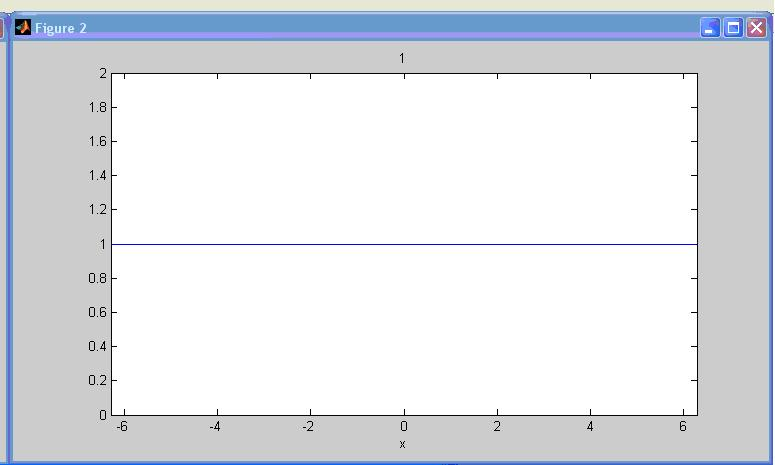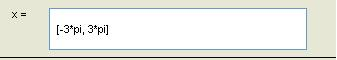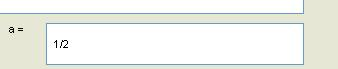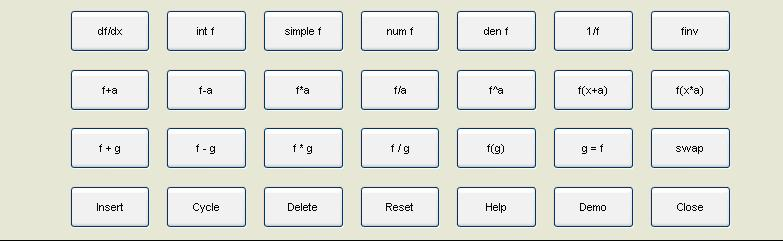BRIGHAM YOUNG UNIVERSITY
 Search BYUContact   |   Help[expand all...]
[COLLAPSE ALL...]# Matlab Tutorial for Beginners

### Written by: Zixu Zhu

Email: sz_jamesrei@hotmail.com
>

# A very convience tool of matlab 7.0 for graphing

If you dont like coding and still want to see the behavior of a function or two functions, the easiest way to do that in MatLab 7.0 is using the funtool function. First, type funtool in the Command Window like this:Then, 3 windows(Figures) will show up:1.Figure 1:
It is the graph of function f which will be defined in Figure 3.2.Figure2:
It is the graph of function g which will also be defined in Figure 3.3.Figure3:a.Function input windows:
f=: type in the expression of f function with variable x, the default expression is x
Example for f=x^2:g=: same as f function, type in the expression of g function , the default expression is 1
Example for g=sin(x):b.Range of x window(x=):
Input the range of x in x= window, the format is [starting value, ending value]
For Example, if we want x from -3pi to 3pi:c.a= window:
This is window is to set the value of a which is a factor of f. It may shift, shrink or enlarge the function f. The default value is ½.d.operation panel
As you see, you can do almost all the common opertions between these two functions or just one function itself. The result plot will show up in Figure 2 or Figure 3.>Maintained by ECEn IMMERSE Web Team.